# Random Tiled

## Recommended Posts

https://goo.gl/SkK44V random tile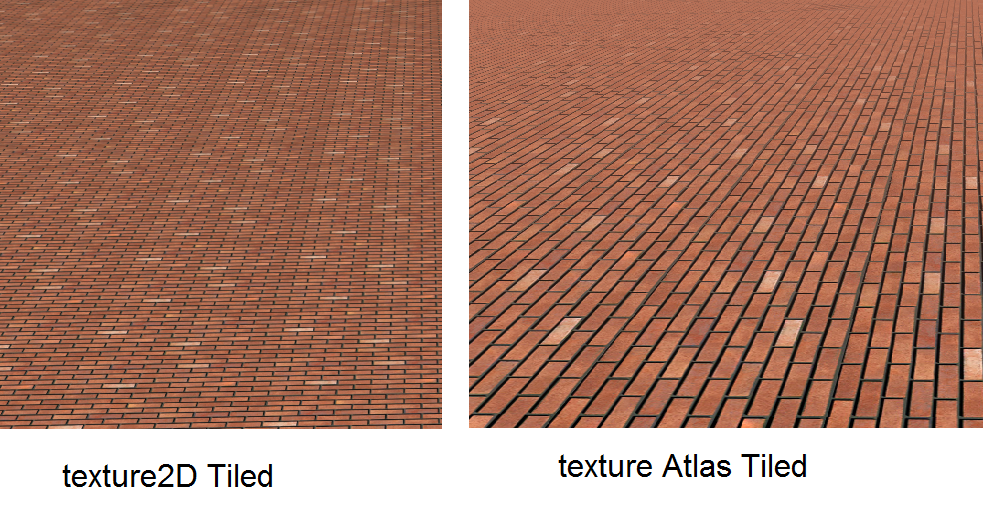main texture for both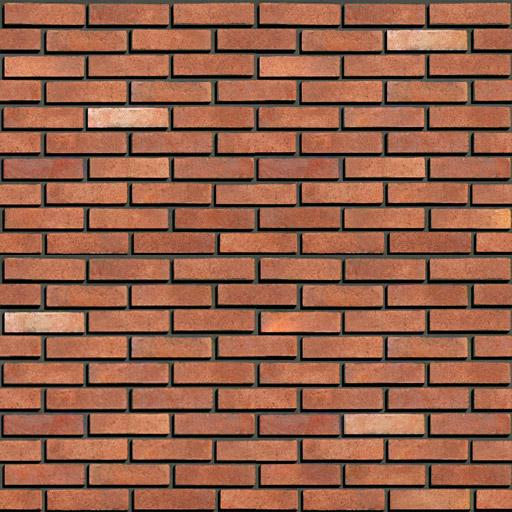##### Share on other sites

excellent !

as usual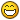##### Share on other sites

On 8/30/2018 at 4:21 AM, Pryme8 said:

is this Synthesized tiling ﻿or just seeded random?

Synthesized with same border not different border

##### Share on other sites

• 2 weeks later...

What a nice idea to random sub tile texture in order to avoid repetitive visual artifacts. ?

I would like to try this tiling out for heightmapped terrain (ground) in my scene.

Is that possible?

Are there any instructions on how to use this?

Does the random tiling also work in combination with mixmaps (3 textures)?

E.g. a grass (9 subtile) texture on green, a rock (9 subtile) texture on red and a snow (9 subtile) texture on blue?

No experience in shaders here... sorry ?

Q

##### Share on other sites

step 1. we need make new UV pattern

``````// setting 1   ( uv.x scale , uv.y scale ,  texture count +1   )
// max texture count for this sample is 9

vec3 r_w1i1 =   vec3(0.033,0.033,10);

// setting 2  (integer seed ,integer seed , float total seed )
// random setting

vec3 r_w2i1 =  vec3(10.,10., 113.321);

// split main texture for calculate new UV

float uxi1 = floor( (vDiffuseUV.x)/r_w1i1.x)*r_w1i1.x;
float uyi1 = floor( vDiffuseUV.y/r_w1i1.y)*r_w1i1.y;

// floor exact uv part to maked random

if(r_w2i1.x != 0. && mod(uyi1 /r_w1i1.y,2.) > 0.)
uxi1 = floor(  (vDiffuseUV.x+r_w1i1.x*r_w2i1.x)  /r_w1i1.x)*r_w1i1.x+r_w1i1.x*0.5 ;
if(r_w2i1.y != 0. && mod(uxi1 /r_w1i1.x,2.) > 0.)
uyi1 = floor(  (vDiffuseUV.y+r_w1i1.y*r_w2i1.y)  /r_w1i1.y)*r_w1i1.y+r_w1i1.y*0.5 ;

// make random fron new uv parts

float rd2i1 = sin(tan(uxi1*r_w2i1.x)+cos(uyi1*r_w2i1.y)*r_w2i1.z+uxi1*r_w2i1.x)*cos(sin(uxi1)*r_w2i1.y+uyi1*r_w2i1.z+sin(r_w2i1.x*tan(uyi1)));

rd2i1 =   (sin(tan(rd2i1*r_w2i1.z)+cos(uyi1*r_w2i1.x)*r_w2i1.y+uxi1*r_w2i1.x)*cos(sin(uxi1)*r_w2i1.z+uyi1*r_w2i1.x+sin(r_w2i1.z*tan(rd2i1)))) ;

rd2i1 =   (sin(tan(rd2i1*r_w2i1.z)+cos(uyi1*r_w2i1.x)*r_w2i1.y+uxi1*r_w2i1.x)*cos(sin(uxi1)*r_w2i1.z+uyi1*r_w2i1.x+sin(r_w2i1.z*tan(rd2i1)))) ;

rd2i1 = floor(min(abs(sin(rd2i1)*r_w1i1.z),r_w1i1.z) )/r_w1i1.z;

// calculate atlas uv pos for 3 x 3 atlas format

float uv_wi1 =   1./3.;

float uv_hi1 =  1./3.;

float dxi1 = 0.;float  dyi1 =0.;

if(rd2i1*r_w1i1.z < 3.) {
dxi1=rd2i1 ;}
else if(rd2i1*r_w1i1.z < 6.)  {
dxi1 = (rd2i1*r_w1i1.z-3.)/r_w1i1.z; dyi1 = 1.; }
else   { dxi1 = (rd2i1*r_w1i1.z-6.)/r_w1i1.z; dyi1 =2.; }

float uv_xi1 =  min(1., dxi1)*r_w1i1.z*1. / 3. ;
float uv_yi1 =  1.-   dyi1*1.  / 3. -uv_hi1;

// claculate new UV

vec2 newUv  = vec2( mod( vDiffuseUV.x *(1./3.)/r_w1i1.x   , uv_wi1 ) +uv_xi1  , mod(vDiffuseUV.y*(1./3.)/r_w1i1.x     ,uv_hi1)+uv_yi1  );

``````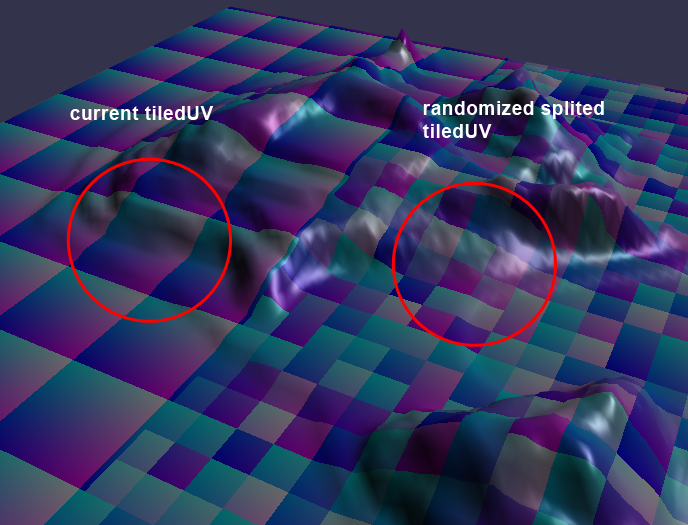##### Share on other sites

step 2 append texture

with this setting  you see just  1, 2 : https://www.babylonjs-playground.com/#FBNKRF#4

vec3 r_w1i1 = vec3(0.05,0.05, );

with this setting  you see just  1, 2,3,4,5,6 : https://www.babylonjs-playground.com/#FBNKRF#5

vec3 r_w1i1 = vec3(0.05,0.05, );

##### Share on other sites

** notic the snow texture is not 3x3 tiled just a simple snow

https://www.babylonjs-playground.com/#FBNKRF#10 with out collision for test fps

##### Share on other sites

Hmmm interesting, looks a lot like how I handled my texture randomisation for my Teriable Generator.

Good job ??

##### Share on other sites

On 9/12/2018 at 9:28 AM, NasimiAsl said:

step 1. we need make new UV pattern

``````// setting 1   ( uv.x scale , uv.y scale ,  texture count +1   )
// max texture count for this sample is 9

vec3 r_w1i1 =   vec3(0.033,0.033,10);

// setting 2  (integer seed ,integer seed , float total seed )
// random setting

vec3 r_w2i1 =  vec3(10.,10., 113.321);

// split main texture for calculate new UV

float uxi1 = floor( (vDiffuseUV.x)/r_w1i1.x)*r_w1i1.x;
float uyi1 = floor( vDiffuseUV.y/r_w1i1.y)*r_w1i1.y;

// floor exact uv part to maked random

if(r_w2i1.x != 0. && mod(uyi1 /r_w1i1.y,2.) > 0.)
uxi1 = floor(  (vDiffuseUV.x+r_w1i1.x*r_w2i1.x)  /r_w1i1.x)*r_w1i1.x+r_w1i1.x*0.5 ;
if(r_w2i1.y != 0. && mod(uxi1 /r_w1i1.x,2.) > 0.)
uyi1 = floor(  (vDiffuseUV.y+r_w1i1.y*r_w2i1.y)  /r_w1i1.y)*r_w1i1.y+r_w1i1.y*0.5 ;

// make random fron new uv parts

float rd2i1 = sin(tan(uxi1*r_w2i1.x)+cos(uyi1*r_w2i1.y)*r_w2i1.z+uxi1*r_w2i1.x)*cos(sin(uxi1)*r_w2i1.y+uyi1*r_w2i1.z+sin(r_w2i1.x*tan(uyi1)));

rd2i1 =   (sin(tan(rd2i1*r_w2i1.z)+cos(uyi1*r_w2i1.x)*r_w2i1.y+uxi1*r_w2i1.x)*cos(sin(uxi1)*r_w2i1.z+uyi1*r_w2i1.x+sin(r_w2i1.z*tan(rd2i1)))) ;

rd2i1 =   (sin(tan(rd2i1*r_w2i1.z)+cos(uyi1*r_w2i1.x)*r_w2i1.y+uxi1*r_w2i1.x)*cos(sin(uxi1)*r_w2i1.z+uyi1*r_w2i1.x+sin(r_w2i1.z*tan(rd2i1)))) ;

rd2i1 = floor(min(abs(sin(rd2i1)*r_w1i1.z),r_w1i1.z) )/r_w1i1.z;

// calculate atlas uv pos for 3 x 3 atlas format

float uv_wi1 =   1./3.;

float uv_hi1 =  1./3.;

float dxi1 = 0.;float  dyi1 =0.;

if(rd2i1*r_w1i1.z < 3.) {
dxi1=rd2i1 ;}
else if(rd2i1*r_w1i1.z < 6.)  {
dxi1 = (rd2i1*r_w1i1.z-3.)/r_w1i1.z; dyi1 = 1.; }
else   { dxi1 = (rd2i1*r_w1i1.z-6.)/r_w1i1.z; dyi1 =2.; }

float uv_xi1 =  min(1., dxi1)*r_w1i1.z*1. / 3. ;
float uv_yi1 =  1.-   dyi1*1.  / 3. -uv_hi1;

// claculate new UV

vec2 newUv  = vec2( mod( vDiffuseUV.x *(1./3.)/r_w1i1.x   , uv_wi1 ) +uv_xi1  , mod(vDiffuseUV.y*(1./3.)/r_w1i1.x     ,uv_hi1)+uv_yi1  );

``````

Thanks

I got this shader code to work for 2x2 (256x256p) and 3x3 (768x768p) subtile textures.
Maybe I create 4x4 also (that will definitely be enough random variations of the same tile).

Perhaps can be parametrized as just another setting "how much subtiles there are" and "how much subtiles you want to use".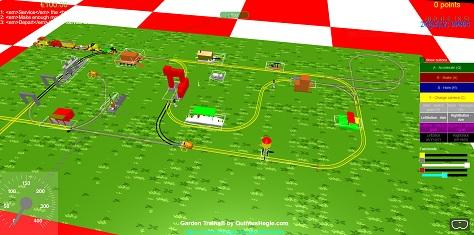^ random grass growing at ground ?

There's a small texture-edge-seam I noticed also in your demo's. I'll leave it for now for my purpose but if you have any ideas on how to remove the visual seam, let us know.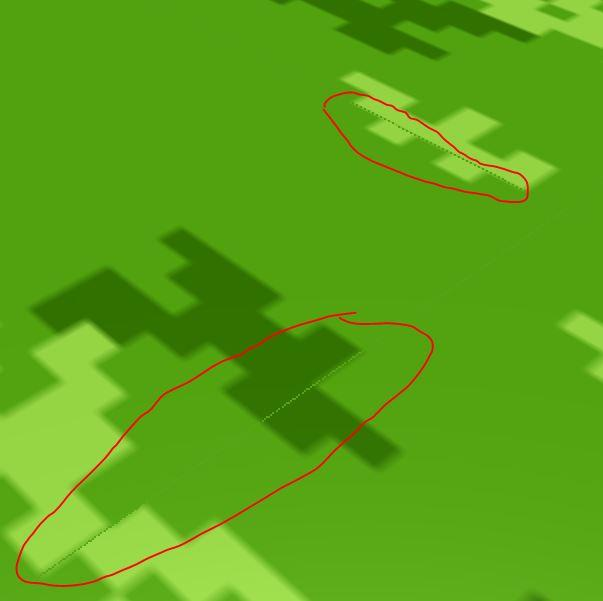Now moving on to your next demo with the mixmap and 3 textures...

This is gonna be awesome ?

Q

##### Share on other sites

let i explain something about texture2D

we cant use exactly this method for atlas map because when that go in far texture2d Mix pixels for make some blur result in far

we can use texture2DLodEXT that not have any mixed pixel if we used lod pametrer 0

but that have other problem in far

in this method we wanna make tile texture so we need all texture be tiled from in edges

but we still have some bug in far*** i cant use texture2DLodEXT in standardMaterial ???

## Join the conversation

You can post now and register later. If you have an account, sign in now to post with your account.
Note: Your post will require moderator approval before it will be visible.×   Pasted as rich text.   Paste as plain text instead

Only 75 emoji are allowed.